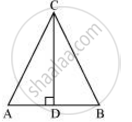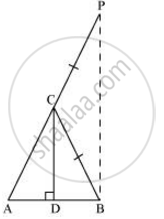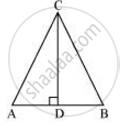# If ∠A and ∠B are acute angles such that cos A = cos B, then show that ∠A = ∠B. - Mathematics

If ∠A and ∠B are acute angles such that cos A = cos B, then show that ∠A = ∠B.

#### Solution

Let us consider a triangle ABC in which CD ⊥ AB.It is given that

cos A = cos B

We have to prove ∠A = ∠B. To prove this, let us extend AC to P such that BC = CP.From equation (1), we obtain

(AB)/(BD) = (AC)/(BC)

=>(AD)/(BD) = (AC)/(CP) (By construction, we have BC = CP ) ....(2)

By using the converse of B.P.T,

CD||BP

⇒∠ACD = ∠CPB (Corresponding angles) … (3)

And, ∠BCD = ∠CBP (Alternate interior angles) … (4)

By construction, we have BC = CP.

∴ ∠CBP = ∠CPB (Angle opposite to equal sides of a triangle) … (5)

From equations (3), (4), and (5), we obtain

∠ACD = ∠BCD … (6)

∠ACD = ∠BCD [Using equation (6)]

∠CDA = ∠CDB [Both 90°]

Therefore, the remaining angles should be equal.

⇒ ∠A = ∠B

Alternatively,

Let us consider a triangle ABC in which CD ⊥ AB.It is given that,

cos A = cos B

=>(AD)/(AC) = (BC)/(BC)

=>(AD)/(BD) = (AC)/(BC)

Let (AD)/(BD) = (AC)/(BC) = k

⇒ AD = k BD … (1)

And, AC = k BC … (2)

Using Pythagoras theorem for triangles CAD and CBD, we obtain

CD2 = AC2 − AD2 … (3)

And, CD2 = BC2 − BD2 … (4)

From equations (3) and (4), we obtain

AC2 − AD2 = BC2 − BD2

⇒ (BC)2 − (k BD)2 = BC2 − BD2

⇒ k2 (BC2 − BD2) = BC2 − BD2

⇒ k2 = 1

⇒ k = 1

Putting this value in equation (2), we obtain

AC = BC

⇒ ∠A = ∠B(Angles opposite to equal sides of a triangle)

Concept: Trigonometric Ratios
Is there an error in this question or solution?

#### APPEARS IN

NCERT Class 10 Maths
Chapter 8 Introduction to Trigonometry
Exercise 8.1 | Q 6 | Page 181